Courses

# Ex 10.4 NCERT Solutions- Circles Class 9 Notes | EduRev

## Class 9 Mathematics by Full Circle

Created by: Full Circle

## Class 9 : Ex 10.4 NCERT Solutions- Circles Class 9 Notes | EduRev

The document Ex 10.4 NCERT Solutions- Circles Class 9 Notes | EduRev is a part of the Class 9 Course Class 9 Mathematics by Full Circle.
All you need of Class 9 at this link: Class 9

Question 1. Two circles of radii 5 cm and 3 cm intersect at two points and the distance between their centres is 4 cm. Find the length of the common chord.
Solution:
We have two intersecting circles with centres at O and O' respectively.
Let PQ be the common chord.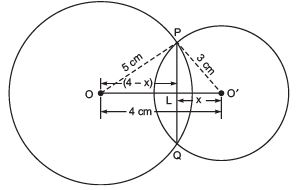∵ In two intersecting circles, the line joining their centres is perpendicular bisector of the common chord.
∴ ∠OLP = ∠OLP = 90º
PL = PQ
Now in right ΔOLP,
PL2 + OL= OP2
⇒ PL2 + (4 - x)2 = 52
⇒ PL= 52 - (4 - x)2
⇒ PL= 25 - 16 - x2 + 8x
⇒ PL2 = 9 - x+ 8x               ...(1)
Again, in ΔO'LP,
PL= 32 - x2 = 9 - x2              ...(2)
From (1) and (2),
we have 9 - x+ 8x = 9 - x2
⇒   8x = 0
⇒   x= 0
⇒ L and O' coincide.
∴ PQ is a diameter of the smaller circle.
⇒ PL = 3 cm
But PL = LQ
∴ LQ = 3 cm
∴ PQ = PL + LQ
= 3 cm + 3 cm = 6 cm
Thus, the required length of the common chord = 6 cm.

Question 2. If two equal chords of a circle intersect within the circle, prove that the segments of one chord are equal to corresponding segments of the other chord.
Solution:
We have a circle with centre O. Equal chords AB and CD intersect at E.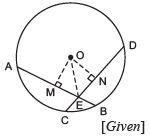To prove that AE = DE and CE = BE,
draw OM ⊥ AB and ON ⊥ CD.
Since AB = CD              [Given]
∴ OM = ON               [Equal chords are equidistant from the centre]
Now, in right ΔOME and right ΔONE,
OM = ON              [Proved]
OE = OE              [Common]
∴ ΔOME ≌ ΔONE              [RHS criterion]
⇒ ME = NE              [c.p.c.t.]
⇒  (1/2) AE = (1/2) DE              [Perpendiculars from the centre bisect the chord.]
⇒ AE = DE               ...(1)
Since AB = CD               (Given)
∴ AB - AE = CD - DE
⇒ CE = BE               ...(2)
From (1) and (2),
we have AE = DE and CE = BE

Question 3. If two equal chords of a circle intersect within the circle, prove that the line joining the point of intersection to the centre makes equal angles with the chords.
Solution:
We have a circle with centre O and equal chords AB and CD are intersecting at E. OE is joined.
To prove that ∠1 = ∠2, let us draw OM ⊥ AB and ON ⊥ CD.
In right ΔOME and right ΔONE,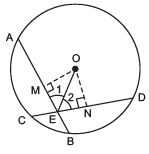OM = ON               [Equal chords are equidistant from the centre.]
OE = OE              [Common]
∴ ΔOME ≌ΔONE              [RHS criterion]
⇒ Their corresponding parts are equal.
∴ ∠OEM = ∠OEN
or ∠OEA = ∠OED

Question 4. If a line intersects two concentric circles (circles with the same centre) with centre O at A, B, C and D, prove that AB = CD (see figure).
Solution:
We have two circles with the common centre O.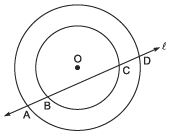A line ‘l’ intersects the outer circle at A and D and the inner circle at B and C. To prove that AB = CD, let us draw OM ⊥ ℓ.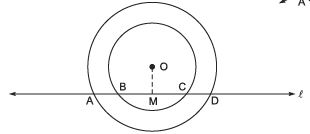For the outer circle,
∵ OM ⊥ ℓ              [Construction]
∴ AM = M D              [∵ Perpendicular from the centre bisects the chord]              ...(1)
For the inner circle,
∵ OM ⊥ ℓ               [Construction]
∴ BM = MC               [Perpendicular from the centre to the chord bisects the chord]              ...(2)
Subtracting (2) from (1),
we have AM - BM = MD - MC
⇒ AB = CD

Question 5. Three girls Reshma, Salma and Mandip are playing a game by standing on a circle of radius 5 m drawn in a park. Reshma throws a ball to Salma, Salma to Mandip, Mandip to Reshma. If the distance between Reshma and Salma and between Salma and Mandip is 6 m each, what is the distance between Reshma and Mandip?
Solution:
Let the three girls Reshma, Salma and Mandip are positioned at R, S and M, respectively on the circle of radius 5 m.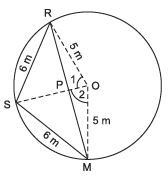∵ RS = SM = 6 m               [Given]
∵ Equal chords of a circle subtend equal angles at the centre.
∴ ∠1 = ∠2
In ΔPOR and ΔPOM,
OP = OP              [Common]
OR = OM              [Radii of the same circle]
∠1= ∠2              [Proved]
∴ ΔPOR ≌ ΔPOM              [SAS criterion]
⇒ Their corresponding parts are equal.
∴ PR = PM and ∠OPR = ∠OPM
∵ ∠OPR + ∠OPM = 180º              [Linear pairs]
∴ ∠OPR = ∠OPM = 90º
⇒ OP ⊥ RM
Now, in ΔRSP and ΔMSP, RS = MS              (6 m each)
SP = SP              (Common)
∠RSP = ∠MSP
∴ ΔRSP ≌ ΔMSP              [SAS criterion]
⇒ RP = MP and ∠RPS = ∠MPS               [c.p.c.t]
But ∠RPS + ∠MPS = 180°
⇒ ∠RPS = ∠MPS = 90º               (Each)
∴ SP passes through O.
Let OP = x m
∴ SP = (5 - x) m
Now, in right ΔOPR, x2 + RP2 = 52               …(1)
In right ΔSPR, (5 - x)2 + RP2 = 62              …(2)
From (1),
RP2 = 52 - x2
From (2),
RP2 = 62 - (5 - x)2
∴ 52 - x2 = 6- (5 - x)2
⇒ 25 - x2 =  36 - [25 - 10x + x2]
⇒ 25 - x2 - 36 + 25 - 10x + x2 = 0
⇒ -10x + 14 = 0
⇒ 10x = 14
⇒ x= (14/10) = 1.4
Now, RP2 = 52 - x
⇒ RP2 = 25 - (1.4)2
⇒ RP2 = 25 - 1.96 = 23.04 m
∴ RP =√(23.04) = 4.8 m
∴ RM = 2 RP = 2 x 4.8 m = 9.6 m
Thus, distance between Reshma and Mandip is 9.6 m.

Question 6. A circular park of radius 20 m is situated in a colony. Three boys Ankur, Syed and David are sitting at equal distance on its boundary each having a toy telephone in his hands to talk each other. Find the length of the string of each phone.
Solution:
In the figure, let Ankur, Syed and David are sitting at A, S and D respectively such that AS = SD = AD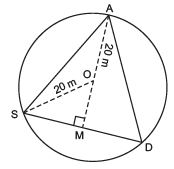i.e.ΔASD is an equilateral triangle.
Let the length of each side of the equilateral triangle is 2x metres.
Let us draw AM ⊥ SD.
Since ΔASD is an equilateral,
∴ AM passes through O.
⇒ SM = (1/2) SD = (1/2) (2x)
⇒ SM = x
Now, in right ΔASM, AM2 + SM2 = AS2
⇒ AM= AS2 - SM2
= (2x)-x2 = 4x2 - x2 = 3x2

⇒ AM = 3x
Now, OM = AM - OA = ( 3x - 20) m
Again, in right ΔOBM, we have
OS= SM2 + OM2
⇒ 202 = x2 + (3x - 20)2
⇒ 400 = x2 + 3x2 - 403 x + 400
⇒ 4x2 - 403 x= 0
⇒ 4x (x - 103) = 0
⇒ x = 0 or x = 103
But x = 0 is not required.
∴ x= 103 m
Now, SD = 2x m = 2 x 103 m
= 203 m.
Thus, the length of the string of each phone = 203 m.

ANGLE SUBTENDED BY AN ARC OF A CIRCLE

REMEMBER

• Equal chords make congruent arcs and congruent arcs make equal chords of a circle.
• If two chords of a circle are equal, then they subtend equal angles at the centre.
• The angle subtended by an arc at the centre is double the angle subtended by it at any point on the remaining part of the circle.
• Angles in the same segment of a circle are equal. Angle in a semicircle is a right angle.
• If a line segment joining two points subtend equal angles at two other points lying on the same side of the line containing the line segment the four points lie on a circle (i.e. they are concyclic).
• A quadrilateral is called cyclic, if all the four vertices of it lie on a circle.
• The sum of either pair of opposite angles of a cyclic quadrilateral is 180°.
• If the sum of a pair of opposite angles of a quadrilateral is 180º, the quadrilateral is cyclic.

,

,

,

,

,

,

,

,

,

,

,

,

,

,

,

,

,

,

,

,

,

;